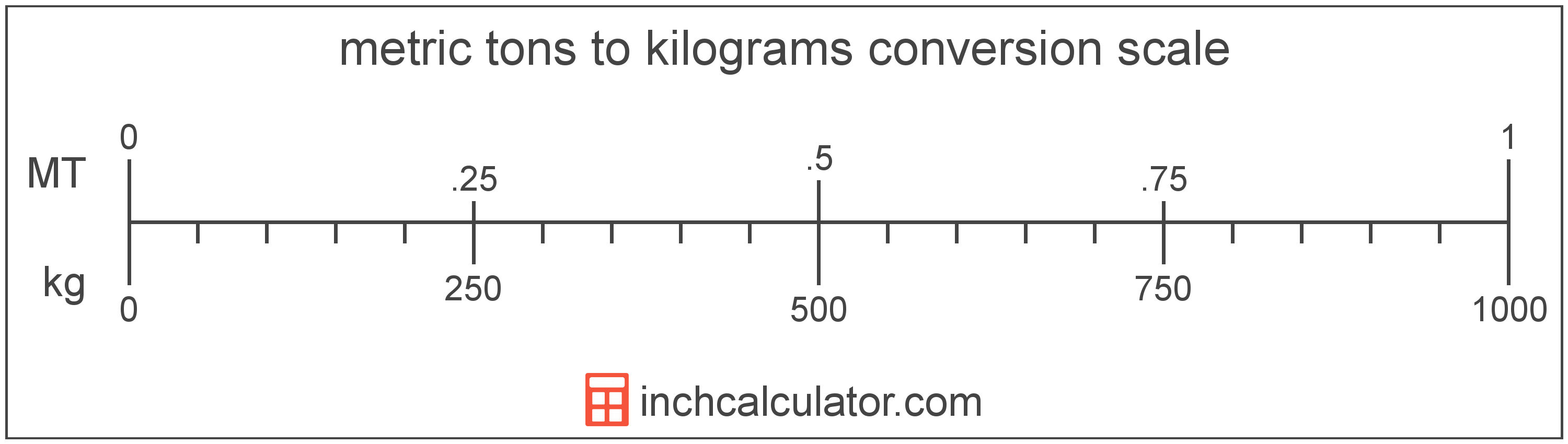# Kilograms to Metric Tons (Tonnes) Conversion

Enter the weight in kilograms below to get the value converted to metric tons.

Results in Metric Tons:1 kg = 0.001 t

## How to Convert Kilograms to Metric TonsTo convert a kilogram measurement to a metric ton measurement, divide the weight by the conversion ratio.

Since one metric ton is equal to 1,000 kilograms, you can use this simple formula to convert:

metric tons = kilograms ÷ 1,000

The weight in metric tons is equal to the kilograms divided by 1,000.

For example, here's how to convert 500 kilograms to metric tons using the formula above.
500 kg = (500 ÷ 1,000) = 0.5 t

## Kilograms

The definition of the kilogram changed in 2019. One kilogram was previously equal to the mass of the platinum-iridium bar, known as the International Prototype of the Kilogram, which was stored in Sèvres, France.

The 2019 SI brochure now defines the kilogram using the Planck constant, and it is defined using the meter and second. It is roughly equal to the mass of 1,000 cubic centimeters of water.

The kilogram, or kilogramme, is the SI base unit for weight and is also a multiple of the gram. In the metric system, "kilo" is the prefix for 103. Kilograms can be abbreviated as kg; for example, 1 kilogram can be written as 1 kg.

## Metric Tons (Tonnes)

One tonne is equal to 1,000 kilograms or 2,204.6 pounds, and is used throughout the world as a unit of mass/weight. One tonne is also equal to 1 megagram. The metric ton should not be confused with short tons, used primarily in the US, or long tons, used primarily in the UK.

The metric ton is an SI accepted unit for weight for use with the metric system. A metric ton is sometimes also referred to as a tonne. Metric tons can be abbreviated as t, and are also sometimes abbreviated as T, Te, or MT. For example, 1 metric ton can be written as 1 t, 1 T, 1 Te, or 1 MT.

In the US the unit is referred to as the metric ton, while the rest of the world refers to it as the tonne. Most often it's still pronounced as tun, but it is sometimes pronounced tunny.

## Kilogram to Metric Ton Conversion Table

Kilogram measurements converted to metric tons
Kilograms Metric Tons
1 kg 0.001 t
2 kg 0.002 t
3 kg 0.003 t
4 kg 0.004 t
5 kg 0.005 t
6 kg 0.006 t
7 kg 0.007 t
8 kg 0.008 t
9 kg 0.009 t
10 kg 0.01 t
20 kg 0.02 t
30 kg 0.03 t
40 kg 0.04 t
50 kg 0.05 t
60 kg 0.06 t
70 kg 0.07 t
80 kg 0.08 t
90 kg 0.09 t
100 kg 0.1 t
200 kg 0.2 t
300 kg 0.3 t
400 kg 0.4 t
500 kg 0.5 t
600 kg 0.6 t
700 kg 0.7 t
800 kg 0.8 t
900 kg 0.9 t
1,000 kg 1 t

## References

1. International Bureau of Weights and Measures, The International System of Units, 9th Edition, 2019, https://www.bipm.org/documents/20126/41483022/SI-Brochure-9-EN.pdf
2. National Institute of Standards and Technology, Specifications, Tolerances, and Other Technical Requirements for Weighing and Measuring Devices, https://www.nist.gov/system/files/documents/2017/05/09/h44-01-all.pdf